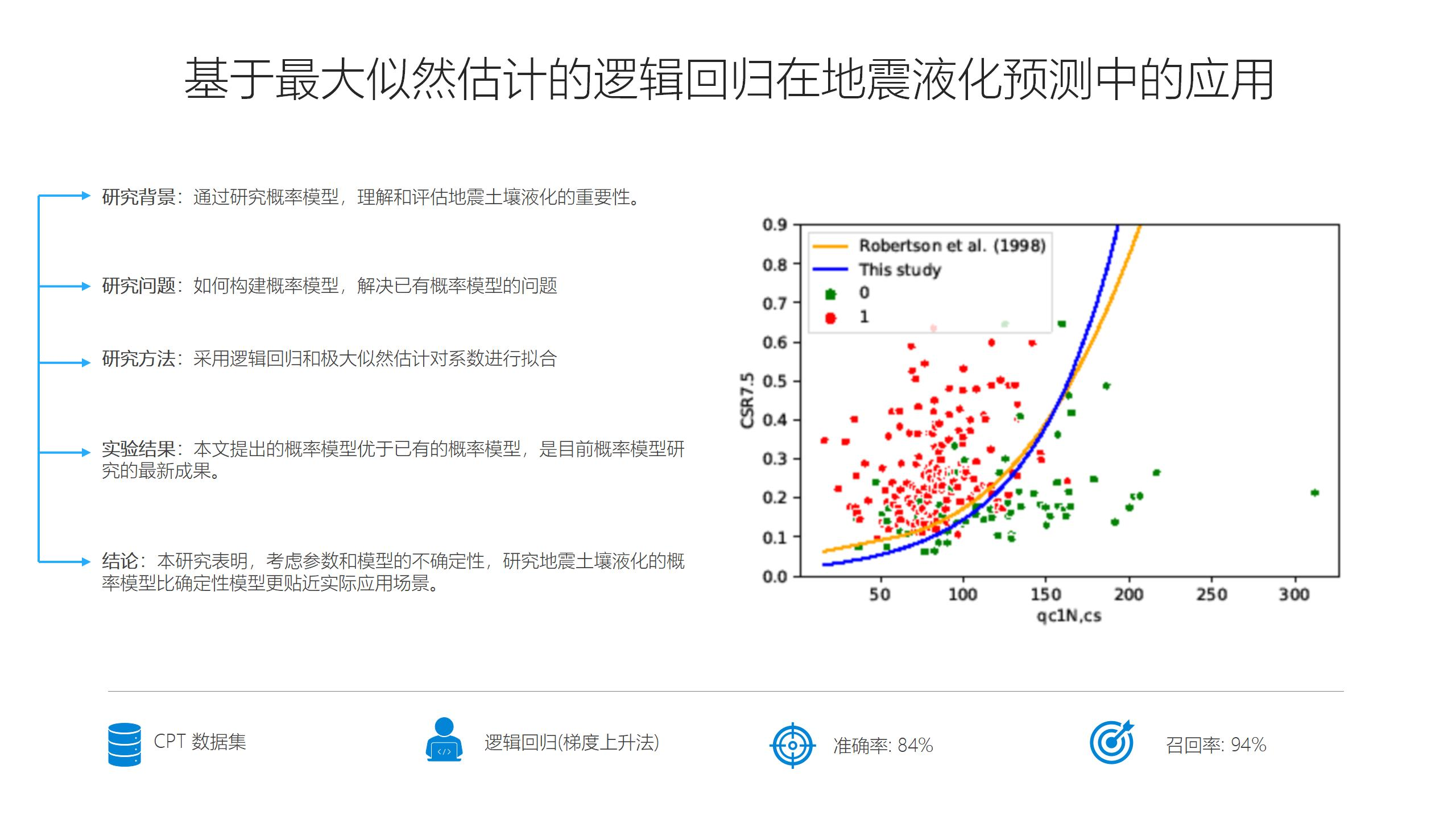Application of Logistic Regression Based on Maximum Likelihood Estimation to Predict Seismic Soil Liquefaction Occurrence2022-01-20Abstract

Seismic soil liquefaction is one of the considerable challenges and disastrous sides of earthquakes that can generally happen in loose to medium saturated sandy soils. The in-situ cone penetration test (CPT) is a widely used index for evaluating the liquefaction characteristics of soils from different sites all over the world. To deal with the uncertainties of the models and the parameters on evaluating the liquefaction, a mathematical probabilistic model is applied via logistic regression, and the comprehensive CPT results are used to develop a model to predict the probability of liquefaction (PL). The new equation to assess the liquefaction occurrence is based on two important features from the expanded CPT dataset. The maximum likelihood estimation (MLE) method is applied to compute the model parameters by maximizing a likelihood function. In addition to that, the sampling bias is applied in the likelihood function via using the weighting factors. Five curve classifiers are plotted for different PL values and ranked using two evaluation metrics. Then, based on these metrics the optimal curve is selected and compared to a well-known deterministic model to validate it. This study also highlights the importance of the recall evaluation metric in the liquefaction occurrence evaluation. The experiment results indicate that the proposed method is outperform existing methods and presents the state-of-the-art in terms of probabilistic models.

Keywords: Probability of liquefaction; logistic regression; classification; maximum likelihood estimation; cone penetration testHuman-Centric Intelligent Systems
ISSN(Online)
2667-1336

2021-12-11

—— Human-Centric Intelligent Systems
—— Human-Centric Intelligent Systems
—— Human-Centric Intelligent Systems